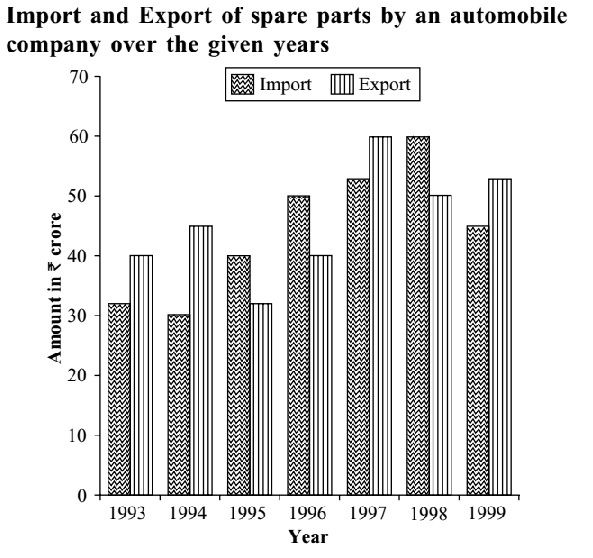## Introduction to Data Interpretation

#### Data Interpretation

Direction: Study the following graph carefully to answer the questions given below it.1. What is the ratio of total imports to total exports for all the given years together?

1. Total imports in the given years = 35 + 30 + 40 + 50 + 55 + 60 + 45 = 315 Million
Total exports in the given years = 40 + 45 + 35 + 40 + 60 + 50 + 55 = 325 Million

##### Correct Option: D

Total imports in the given years = 35 + 30 + 40 + 50 + 55 + 60 + 45 = 315 Million
Total exports in the given years = 40 + 45 + 35 + 40 + 60 + 50 + 55 = 325 Million
Hence, required ratio =

 315 = 63 325 65

1. The total exports in the years 1995, 1996 and 1999 together are what % of the total import during the same period? (up to two decimal places)

1. Total exports in the years 1995, 1996 and 1999 = 35 + 40 + 55 = 130 Million
Total imports in the years 1995, 1996 and 1999 = 40 + 50 + 45 = 135 Million.

##### Correct Option: E

Total exports in the years 1995, 1996 and 1999 = 35 + 40 + 55 = 130 Million
Total imports in the years 1995, 1996 and 1999 = 40 + 50 + 45 = 135 Million.

 Now, Required % = 130 X 100 = 96.29 % 135

1. Which of the following pairs of years and the % increase in the export over the previous year is correctly matched?

1. If you calculate approximate value you reject (b), (c) and (d).
Now check option (a).
In 1996 , calculate % increase in export

##### Correct Option: A

If you calculate approximate value you reject (b), (c) and (d). Now check (a).
In 1996, % increase in export

 = 5 x 100 = 100 = 14.29% 35 7

1. During which year the percentage rise/fall in imports from the previous year is the lowest?

1. As per given graph we can see the difference is very less in 1998.

##### Correct Option: B

As per given graph we can see the difference is very less in 1998. Obvious from the chart its 1998 .

1. In which of the following pairs of years the total import is equal to total export in the same pair of years?

1. See the chart and compare the graph.

##### Correct Option: C

Obvious from the chart , we can say that 1998–1999 year has the same import and export.